## An R Companion for the Handbook of Biological Statistics

Salvatore S. Mangiafico

# Two-way Anova

### Packages used in this chapter

The following commands will install these packages if they are not already installed:

if(!require(FSA)){install.packages("FSA")}
if(!require(ggplot2)){install.packages("ggplot2")}
if(!require(car)){install.packages("car")}
if(!require(multcompView)){install.packages("multcompView")}
if(!require(lsmeans)){install.packages("lsmeans")}
if(!require(grid)){install.packages("grid")}
if(!require(nlme)){install.packages("nlme")}
if(!require(lme4)){install.packages("lme4")}
if(!require(lmerTest)){install.packages("lmerTest")} if(!require(rcompanion)){install.packages("rcompanion")}

When to use it

Null hypotheses

How the test works

Assumptions

See the Handbook for information on these topics.

Examples

The rattlesnake example is shown at the end of the “How to do the test” section.

### How to do the test

For notes on linear models and conducting anova, see the “How to do the test” section in the One-way anova chapter of this book.  For two-way anova with robust regression, see the chapter on Two-way Anova with Robust Estimation.

#### Two-way anova example

### --------------------------------------------------------------
### Two-way anova, SAS example, pp. 179–180
### --------------------------------------------------------------

Input = ("
id Sex     Genotype  Activity
1 male    ff        1.884
2 male    ff        2.283
3 male    fs        2.396
4 female  ff        2.838
5 male    fs        2.956
6 female  ff        4.216
7 female  ss        3.620
8 female  ff        2.889
9 female  fs        3.550
10 male    fs        3.105
11 female  fs        4.556
12 female  fs        3.087
13 male    ff        4.939
14 male    ff        3.486
15 female  ss        3.079
16 male    fs        2.649
17 female  fs        1.943
19 female  ff        4.198
20 female  ff        2.473
22 female  ff        2.033
24 female  fs        2.200
25 female  fs        2.157
26 male    ss        2.801
28 male    ss        3.421
29 female  ff        1.811
30 female  fs        4.281
32 female  fs        4.772
34 female  ss        3.586
36 female  ff        3.944
38 female  ss        2.669
39 female  ss        3.050
41 male    ss        4.275
43 female  ss        2.963
46 female  ss        3.236
48 female  ss        3.673
49 male    ss        3.110
")

##### Means and summary statistics by group

library(Rmisc)

sum = summarySE(Data,
measurevar="Activity",
groupvars=c("Sex","Genotype"))
sum

Sex Genotype N Activity        sd        se        ci

1 female       ff 8  3.05025 0.9599032 0.3393770 0.8024992

2 female       fs 8  3.31825 1.1445388 0.4046556 0.9568584

3 female       ss 8  3.23450 0.3617754 0.1279069 0.3024518

4   male       ff 4  3.14800 1.3745115 0.6872558 2.1871546

5   male       fs 4  2.77650 0.3168433 0.1584216 0.5041684

6   male       ss 4  3.40175 0.6348109 0.3174055 1.0101258

##### Interaction plot using summary statistics

library(ggplot2)

pd = position_dodge(.2)

ggplot(sum, aes(x=Genotype,
y=Activity,
color=Sex)) +
geom_errorbar(aes(ymin=Activity-se,
ymax=Activity+se),
width=.2, size=0.7, position=pd) +
geom_point(shape=15, size=4, position=pd) +
theme_bw() +
theme(
axis.title.y = element_text(vjust= 1.8),
axis.title.x = element_text(vjust= -0.5),
axis.title = element_text(face = "bold")) +
scale_color_manual(values = c("black", "blue"))

### You may see an error, “ymax not defined”
###  In this case, it does not appear to affect anythingInteraction plot for a two-way anova.  Square points represent means for groups, and error bars indicate standard errors of the mean.

##### Simple box plot of main effect and interaction

boxplot(Activity ~ Genotype,
data = Data,
xlab = "Genotype",
ylab = "MPI Activity")boxplot(Activity ~ Genotype:Sex,
data = Data,
xlab = "Genotype x Sex",
ylab = "MPI Activity")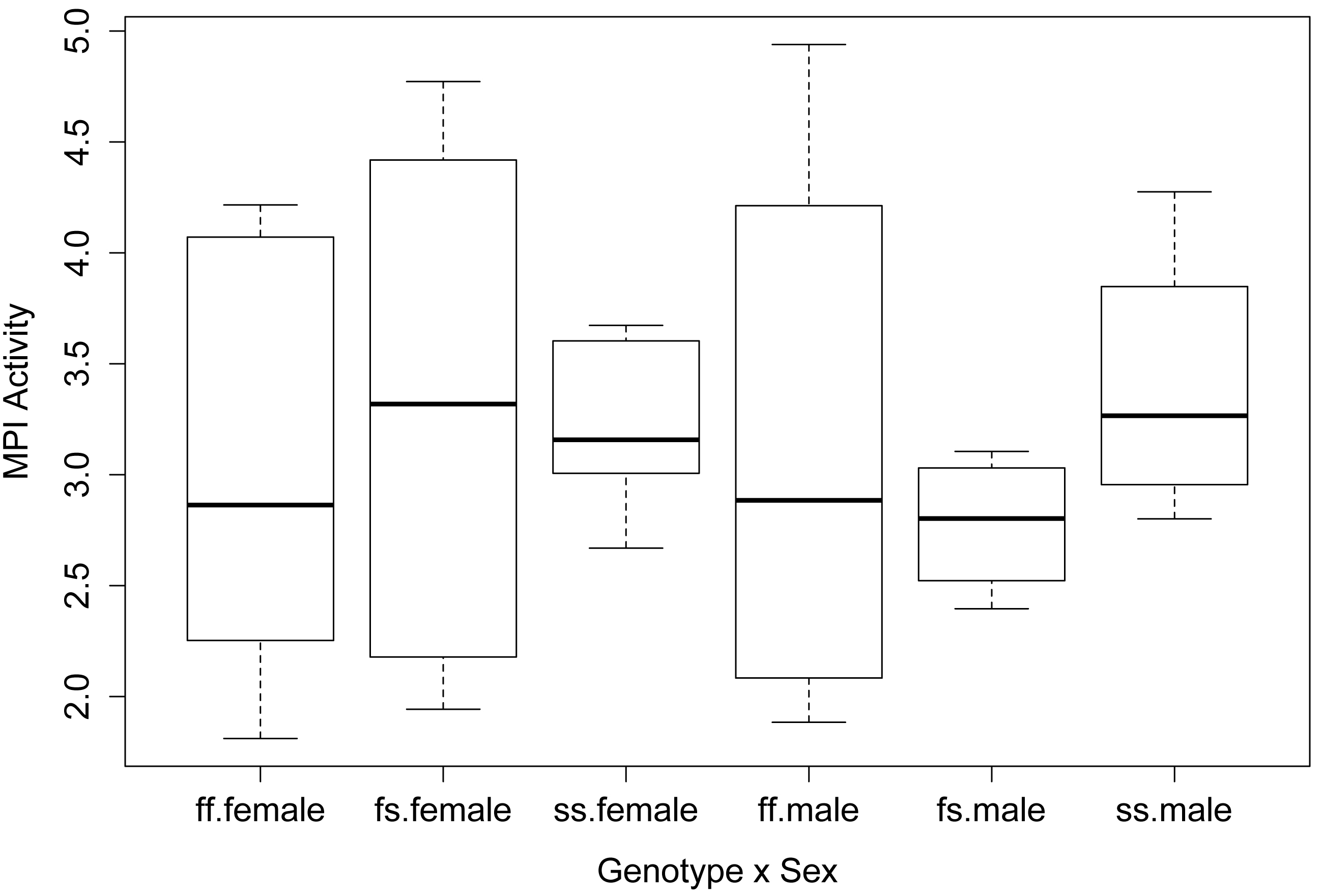##### Fit the linear model and conduct ANOVA

model = lm(Activity ~ Sex + Genotype + Sex:Genotype,
data=Data)

library(car)

Anova(model, type="II")                    # Can use type="III"

### If you use type="III", you need the following line before the analysis
###
options(contrasts = c("contr.sum", "contr.poly"))

Sum Sq Df F value Pr(>F)

Sex           0.0681  1  0.0861 0.7712

Genotype      0.2772  2  0.1754 0.8400

Sex:Genotype  0.8146  2  0.5153 0.6025

Residuals    23.7138 30

anova(model)                               # Produces type I sum of squares

Df  Sum Sq Mean Sq F value Pr(>F)

Sex           1  0.0681 0.06808  0.0861 0.7712

Genotype      2  0.2772 0.13862  0.1754 0.8400

Sex:Genotype  2  0.8146 0.40732  0.5153 0.6025

Residuals    30 23.7138 0.79046

summary(model)     # Produces r-square, overall p-value, parameter estimates

Multiple R-squared:  0.04663, Adjusted R-squared:  -0.1123

F-statistic: 0.2935 on 5 and 30 DF,  p-value: 0.9128

##### Checking assumptions of the model

hist(residuals(model),
col="darkgray")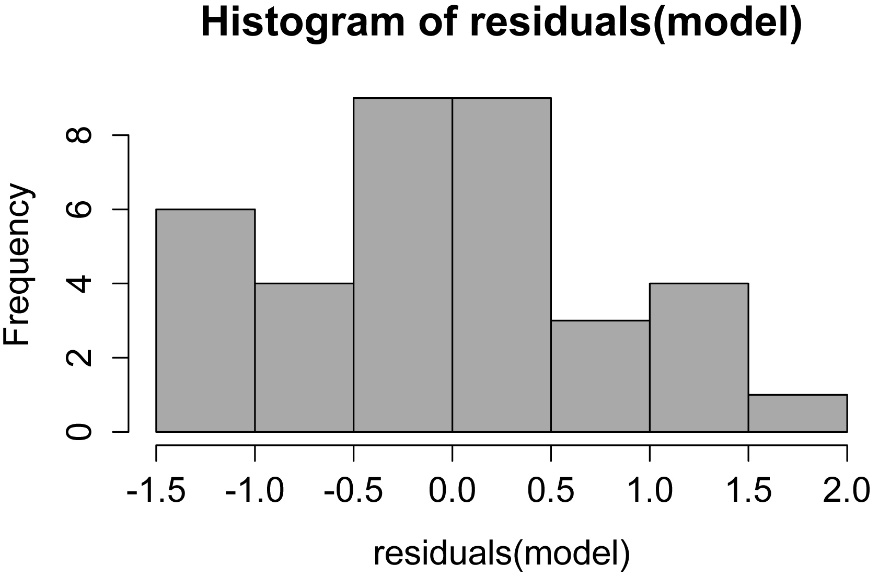A histogram of residuals from a linear model.  The distribution of these residuals should be approximately normal.

plot(fitted(model),
residuals(model))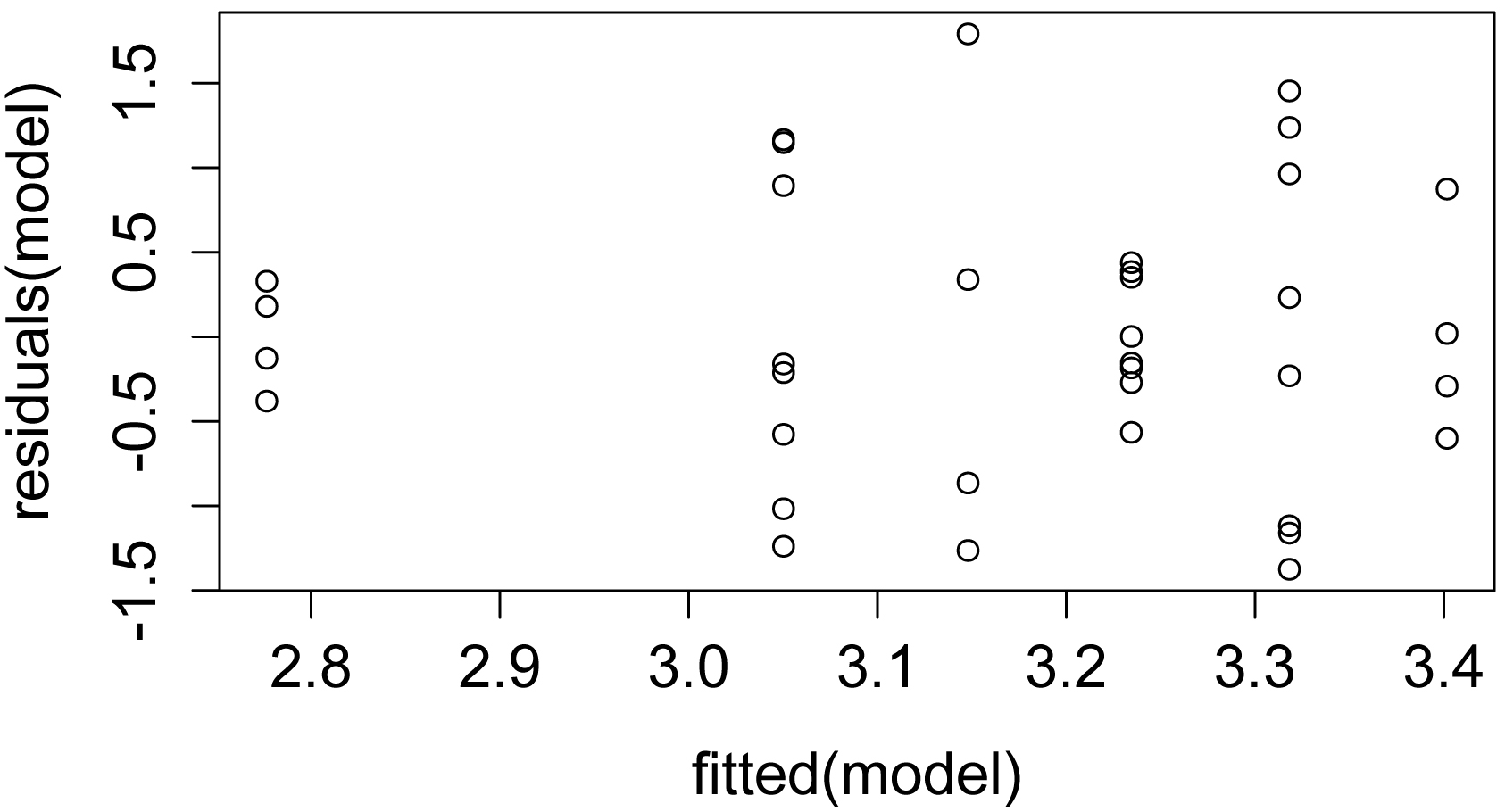A plot of residuals vs. predicted values.  The residuals should be unbiased and homoscedastic.  For an illustration of these properties, see this diagram by Steve Jost at DePaul University: condor.depaul.edu/sjost/it223/documents/resid-plots.gif.

### additional model checking plots with: plot(model)
### alternative: library(FSA); residPlot(model)

#### Post-hoc comparison of least-square means

For notes on least-square means, see the “Post-hoc comparison of least-square means” section in the Nested anova chapter in this book.

One advantage of the using the lsmeans package for post-hoc tests is that it can produce comparisons for interaction effects.

In general, if the interaction effect is significant, you will want to look at comparisons of means for the interactions.  If the interaction effect is not significant but a main effect is, it is appropriate to look at comparisons among the means for that main effect.  In this case, because no effect of Sex, Genotype, or Sex:Genotype was significant, we would not actually perform any mean separation test.

##### Mean separations for main factor with lsmeans

library(multcompView)
library(lsmeans)

lsmeans = lsmeans::lsmeans ### Uses the lsmeans function
###  from the lsmeans package,
###  not from the lmerTest package

leastsquare = lsmeans(model,
"Genotype",

cld(leastsquare,
alpha=.05,
Letters=letters)

Genotype   lsmean        SE df lower.CL upper.CL .group

fs       3.047375 0.2722236 30 2.359065 3.735685  a

ff       3.099125 0.2722236 30 2.410815 3.787435  a

ss       3.318125 0.2722236 30 2.629815 4.006435  a

### Means sharing a letter in .group are not significantly different

##### Mean separations for interaction effect with lsmeans

library(multcompView)
library(lsmeans)

lsmeans = lsmeans::lsmeans ### Uses the lsmeans function
###  from the lsmeans package,
###  not from the lmerTest package

leastsquare = lsmeans(model,
pairwise ~ Sex:Genotype,

cld(leastsquare,
alpha=.05,
Letters=letters)

Sex    Genotype  lsmean        SE df lower.CL upper.CL .group

male   fs       2.77650 0.4445393 30 1.524666 4.028334  a

female ff       3.05025 0.3143368 30 2.165069 3.935431  a

male   ff       3.14800 0.4445393 30 1.896166 4.399834  a

female ss       3.23450 0.3143368 30 2.349319 4.119681  a

female fs       3.31825 0.3143368 30 2.433069 4.203431  a

male   ss       3.40175 0.4445393 30 2.149916 4.653584  a

### Note that means are listed from low to high,

###  not in the same order as summarySE

#### Graphing the results

##### Simple bar plot with categories and no error bars

### Re-enter data as matrix

Input =("
Sex      ff       fs       ss
Female  3.05025  3.31825  3.23450
Male    3.14800  2.77650  3.40175
")

row.names=1))

Matriz

ff      fs      ss

Female 3.05025 3.31825 3.23450

Male   3.14800 2.77650 3.40175

barplot(Matriz,
beside=TRUE,
legend=TRUE,
ylim=c(0, 5),
xlab="Genotype",
ylab="MPI Activity")##### Bar plot with error bars with ggplot2

This plot uses the data frame created by summarySE in Rmisc.  Error bars indicate standard error of the means (se in the data frame).

library(Rmisc)

sum = summarySE(Data, measurevar="Activity", groupvars=c("Sex","Genotype"))

sum

Sex Genotype N Activity        sd        se        ci

1 female       ff 8  3.05025 0.9599032 0.3393770 0.8024992

2 female       fs 8  3.31825 1.1445388 0.4046556 0.9568584

3 female       ss 8  3.23450 0.3617754 0.1279069 0.3024518

4   male       ff 4  3.14800 1.3745115 0.6872558 2.1871546

5   male       fs 4  2.77650 0.3168433 0.1584216 0.5041684

6   male       ss 4  3.40175 0.6348109 0.3174055 1.0101258

###   shinyapps.stat.ubc.ca/r-graph-catalog/

library(ggplot2)
library(grid)

ggplot(sum,
aes(x = Genotype, y = Activity, fill = Sex,
ymax=Activity+se, ymin=Activity-se))  +
geom_bar(stat="identity", position = "dodge", width = 0.7) +
geom_bar(stat="identity", position = "dodge",
colour = "black", width = 0.7,
show_guide = FALSE)  +
scale_y_continuous(breaks = seq(0, 4, 0.5),
limits = c(0, 4),
expand = c(0, 0))  +
scale_fill_manual(name = "Count type" ,
values = c('grey80', 'grey30'),
labels = c("Female",
"Male"))  +
geom_errorbar(position=position_dodge(width=0.7),
width=0.0, size=0.5, color="black")  +
labs(x = "Genotype",
y = "MPI Activity")  +
## ggtitle("Main title") +
theme_bw()  +
theme(panel.grid.major.x = element_blank(),
panel.grid.major.y = element_line(colour = "grey50"),
plot.title = element_text(size = rel(1.5),
face = "bold", vjust = 1.5),
axis.title = element_text(face = "bold"),
legend.position = "top",
legend.title = element_blank(),
legend.key.size = unit(0.4, "cm"),
legend.key = element_rect(fill = "black"),
axis.title.y = element_text(vjust= 1.8),
axis.title.x = element_text(vjust= -0.5)
)

#     #     #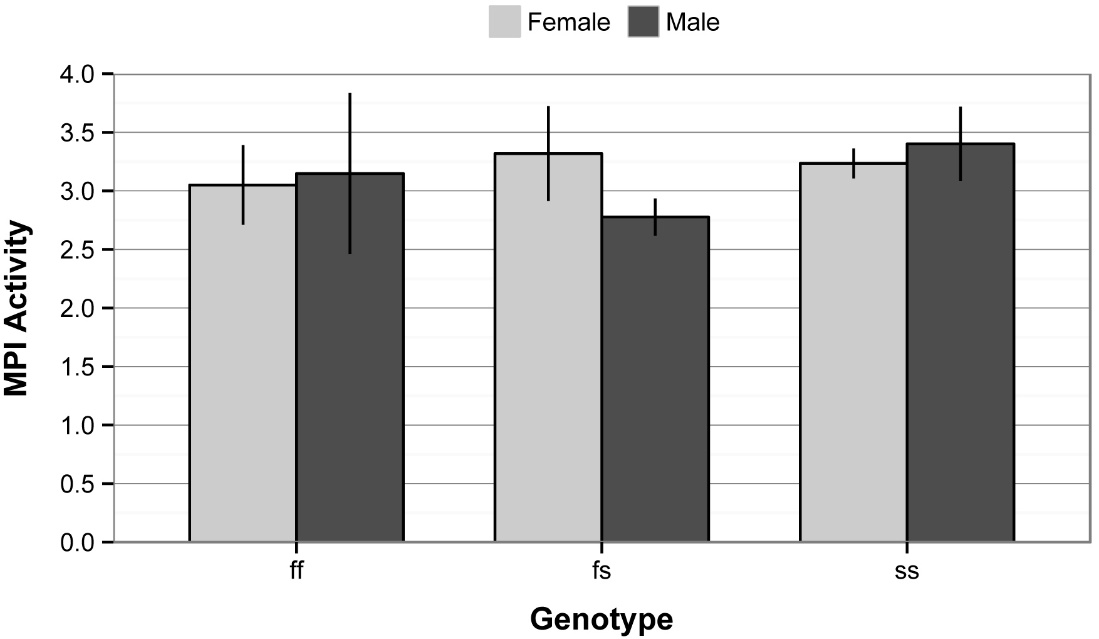Bar plot for a two-way anova.  Bar heights represent means for groups, and error bars indicate standard errors of the mean.

#### Rattlesnake example – two-way anova without replication, repeated measures

This example could be interpreted as two-way anova without replication or as a one-way repeated measures experiment. Below it is analyzed as a two-way fixed effects model using the lm function, and as a mixed effects model using the nlme package and lme4 packages.

### --------------------------------------------------------------
### Two-way anova, rattlesnake example, pp. 177–178
### --------------------------------------------------------------

Input = ("
Day  Snake  Openings
1    D1        85
1    D3       107
1    D5        61
1    D8        22
1    D11       40
1    D12       65
2    D1        58
2    D3        51
2    D5        60
2    D8        41
2    D11       45
2    D12       27
3    D1        15
3    D3        30
3    D5        68
3    D8        63
3    D11       28
3    D12        3
4    D1        57
4    D3        12
4    D5        36
4    D8        21
4    D11       10
4    D12       16
")

### Treat Day as a factor variable

Data\$Day = as.factor(Data\$Day)

#### Using two-way fixed effects model

##### Means and summary statistics by group

library(Rmisc)

sum = summarySE(Data, measurevar="Openings", groupvars=c("Day"))

sum

Day N Openings       sd        se       ci

1   1 6 63.33333 30.45434 12.432931 31.95987

2   2 6 47.00000 12.21475  4.986649 12.81859

3   3 6 34.50000 25.95958 10.597956 27.24291

4   4 6 25.33333 18.08498  7.383164 18.97903

##### Simple box plots

boxplot(Openings ~ Day,
data = Data,
xlab = "Day",
ylab = "Openings until tail stops rattling")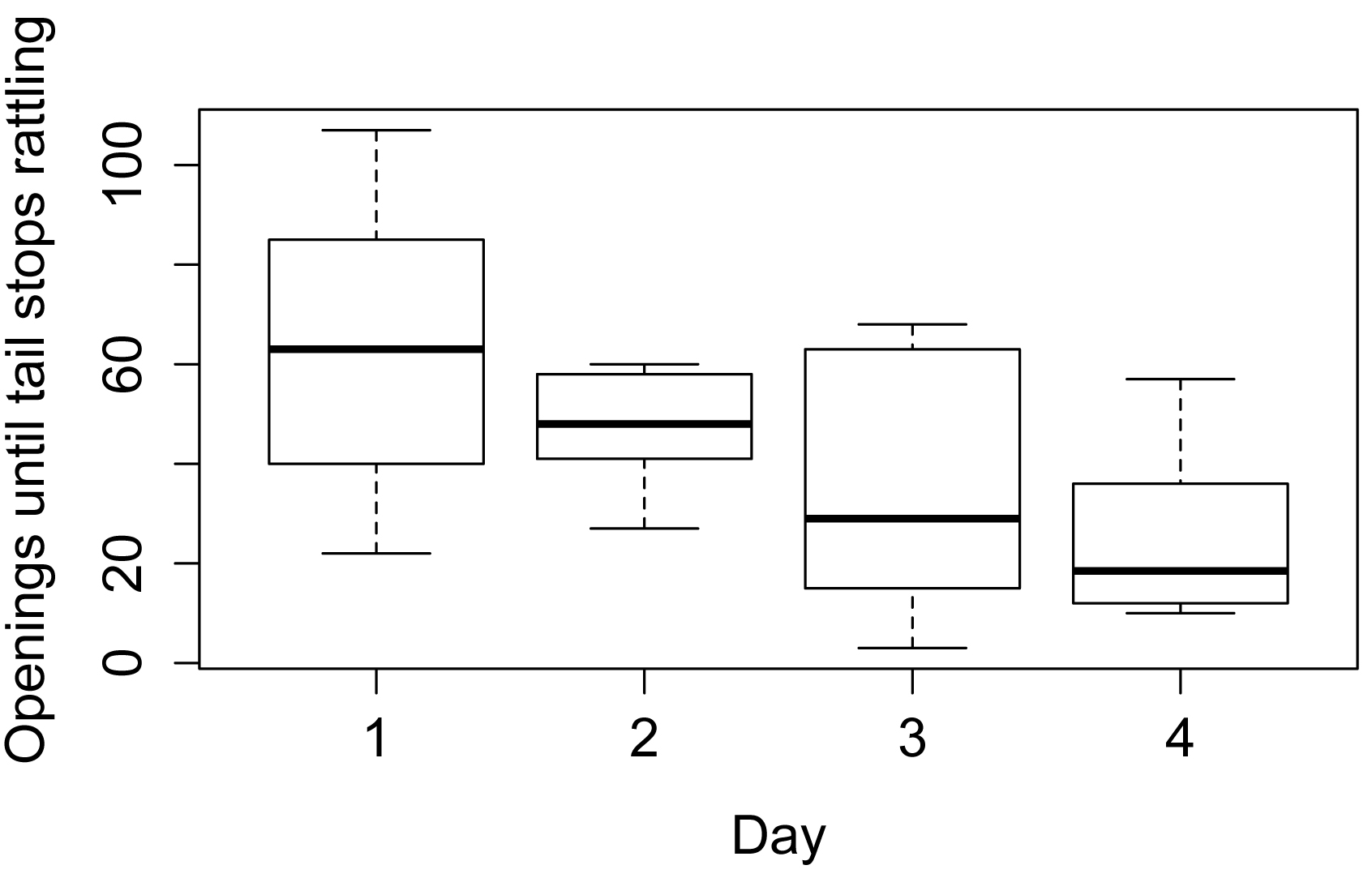##### Fit the linear model and conduct ANOVA

model = lm(Openings ~ Day + Snake,
data=Data)

library(car)

Anova(model, type="II")                    # Can use type="III"

Sum Sq Df F value  Pr(>F)

Day       4877.8  3  3.3201 0.04866 *

Snake     3042.2  5  1.2424 0.33818

Residuals 7346.0 15

anova(model)                               # Produces type I sum of squares

Df Sum Sq Mean Sq F value  Pr(>F)

Day        3 4877.8 1625.93  3.3201 0.04866 *

Snake      5 3042.2  608.44  1.2424 0.33818

Residuals 15 7346.0  489.73

summary(model)     # Produces r-square, overall p-value, parameter estimates

Multiple R-squared:  0.5188,  Adjusted R-squared:  0.2622

F-statistic: 2.022 on 8 and 15 DF,  p-value: 0.1142

##### Checking assumptions of the model

hist(residuals(model),
col="darkgray")A histogram of residuals from a linear model.  The distribution of these residuals should be approximately normal.

plot(fitted(model),
residuals(model))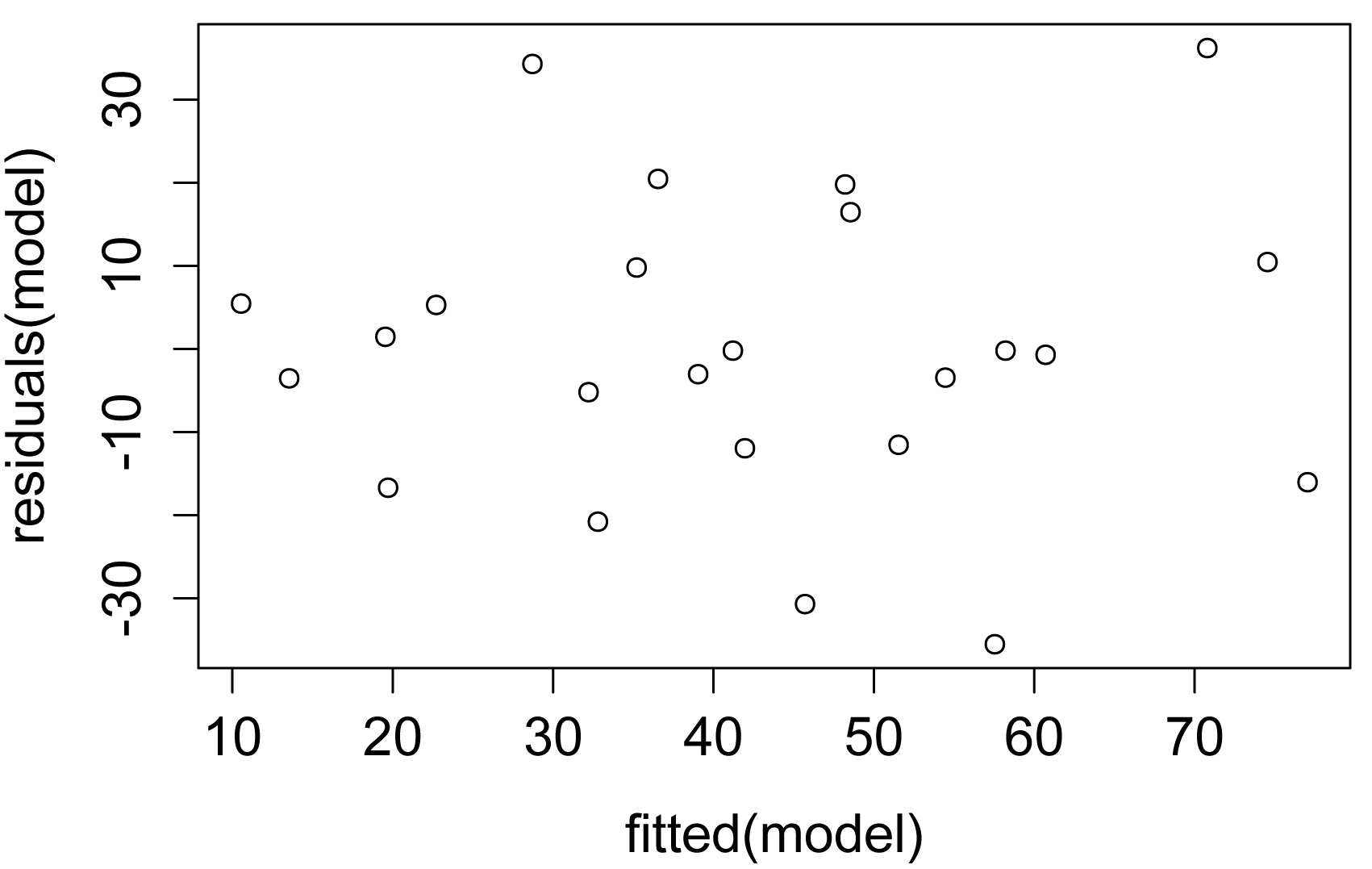A plot of residuals vs. predicted values.  The residuals should be unbiased and homoscedastic.  For an illustration of these properties, see this diagram by Steve Jost at DePaul University: condor.depaul.edu/sjost/it223/documents/resid-plots.gif.

### additional model checking plots with: plot(model)
### alternative: library(FSA); residPlot(model)

##### Mean separations for main factor with lsmeans

For notes on least-square means, see the “Post-hoc comparison of least-square” means section in the Nested anova chapter in this book.  For other mean separation techniques for a main factor in anova, see “Tukey and Least Significant Difference mean separation tests (pairwise comparisons)” section in the One-way anova chapter.

library(multcompView)
library(lsmeans)

lsmeans = lsmeans::lsmeans ### Uses the lsmeans function
###  from the lsmeans package,
###  not from the lmerTest package

leastsquare = lsmeans(model,
"Day",

cld(leastsquare,
alpha=.05,
Letters=letters)

Day   lsmean       SE df   lower.CL upper.CL .group

4   25.33333 9.034476 15 -0.2085871 50.87525  a

3   34.50000 9.034476 15  8.9580796 60.04192  ab

2   47.00000 9.034476 15 21.4580796 72.54192  ab

1   63.33333 9.034476 15 37.7914129 88.87525   b

### Means sharing a letter in .group are not significantly different

#### Using mixed effects model with nlme

This is an abbreviated example using the lme function in the nlme package.

library(nlme)

model = lme(Openings ~ Day, random=~1|Snake,
data=Data,
method="REML")

anova.lme(model,
type="sequential",

numDF denDF  F-value p-value
(Intercept)     1    15 71.38736  <.0001
Day             3    15  3.32005  0.0487

library(multcompView)
library(lsmeans)

lsmeans = lsmeans::lsmeans ### Uses the lsmeans function
###  from the lsmeans package,
###  not from the lmerTest package

leastsquare = lsmeans(model,
"Day",

cld(leastsquare,
alpha=.05,
Letters=letters)

Day   lsmean       SE df asymp.LCL asymp.UCL .group
4   25.33333 9.304196 NA  2.157372  48.50929  a
3   34.50000 9.304196 NA 11.324038  57.67596  ab
2   47.00000 9.304196 NA 23.824038  70.17596  ab
1   63.33333 9.304196 NA 40.157372  86.50929   b

### Means sharing a letter in .group are not significantly different

#### Using mixed effects model with lmer

This is an abbreviated example using the lmer function in the lme4 package.

library(lme4)
library(lmerTest)

model = lmer(Openings ~ Day + (1|Snake),
data=Data,
REML=TRUE)

anova(model)

Analysis of Variance Table of type III  with  Satterthwaite
approximation for degrees of freedom

Sum Sq Mean Sq NumDF DenDF F.value  Pr(>F)
Day 4877.8  1625.9     3    15  3.3201 0.04866 *

rand(model)

Analysis of Random effects Table:
Chi.sq Chi.DF p.value
Snake 0.0915      1     0.8

##### Least square means with the lsmeans package

library(multcompView)
library(lsmeans)

lsmeans = lsmeans::lsmeans ### Uses the lsmeans function
###  from the lsmeans package,
###  not from the lmerTest package

leastsquare = lsmeans(model,
pairwise ~ Day,
alpha=.05,

cld(leastsquare,
alpha=.05,
Letters=letters,

Day   lsmean       SE    df   lower.CL upper.CL .group
4   25.33333 9.304196 19.81 -0.1441779 50.81084  a
3   34.50000 9.304196 19.81  9.0224887 59.97751  ab
2   47.00000 9.304196 19.81 21.5224887 72.47751  ab
1   63.33333 9.304196 19.81 37.8558221 88.81084   b

Degrees-of-freedom method: satterthwaite
Confidence level used: 0.95
Conf-level adjustment: sidak method for 4 estimates
P value adjustment: tukey method for comparing a family of 4 estimates
significance level used: alpha = 0.05

### Means sharing a letter in .group are not significantly different

##### Least square means using the lmerTest package

lsmeans = lmerTest::lsmeans ### Uses the lsmeans function
###  from the lmerTest package,
###  not from the lsmeans package

LT = lsmeans(model,
test.effs = "Day")

LT

Least Squares Means table:
Day Estimate Standard Error   DF t-value Lower CI Upper CI p-value
Day  1  1.0    63.33           9.30 19.8    6.81    43.91     82.8  <2e-16 ***
Day  2  2.0    47.00           9.30 19.8    5.05    27.58     66.4   1e-04 ***
Day  3  3.0    34.50           9.30 19.8    3.71    15.08     53.9   0.001 **
Day  4  4.0    25.33           9.30 19.8    2.72     5.91     44.8   0.013 *

PT = difflsmeans(model,
test.effs="Day")

PT

Differences of LSMEANS:

Estimate Standard Error   DF t-value Lower CI Upper CI p-value
Day 1 - 2     16.3          12.78 15.0    1.28   -10.90     43.6   0.220
Day 1 - 3     28.8          12.78 15.0    2.26     1.60     56.1   0.039 *
Day 1 - 4     38.0          12.78 15.0    2.97    10.77     65.2   0.009 **
Day 2 - 3     12.5          12.78 15.0    0.98   -14.73     39.7   0.343
Day 2 - 4     21.7          12.78 15.0    1.70    -5.57     48.9   0.111
Day 3 - 4      9.2          12.78 15.0    0.72   -18.07     36.4   0.484

### Extract lsmeans table

Sum = PT\$diffs.lsmeans.table

### Extract comparisons and p-values

Comparison = rownames(Sum)

P.value   = Sum\$'p-value'

method =
"none")

### Fix names of comparisons

Comparison = gsub(
"-", "- Day", Comparison)

### Produce compact letter display

library(rcompanion)

cldList(comparison = Comparison,